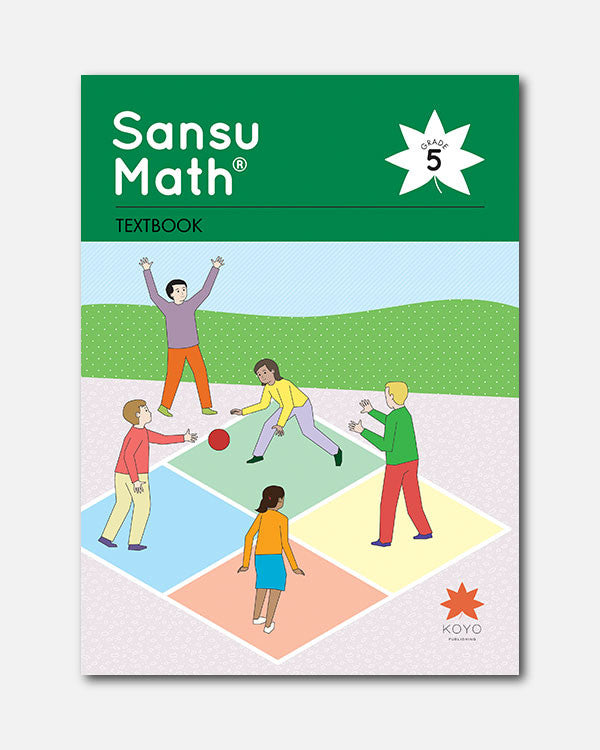# Sansu Math® Textbook 5

In Stock
\$ 25.00

### Overview

Sansu Math® Grade 5 is the final book in the Sansu Math® elementary mathematics series. The material includes 15 units in one textbook, which includes the same student and shape characters used throughout the series.

1. Whole Numbers and Decimal Numbers
2. Volume of Cubes and Cuboids
1. How to Express the Amount of Space
2. Various Units of Volume
3. Multiplication of Decimal Numbers
1. Multiplication of Decimal Numbers
2. Times as Much and Decimal Numbers
4. Division of Decimal Numbers
1. Division of Decimal Numbers
2. Division and Times as Much with Decimal Numbers
5. Congruent Shapes
6. Even and Odd Numbers, Multiples and Factors
1. Even and Odd Numbers
2. Multiples and Common Multiples
3. Factors and Common Factors
7. Per Unit Quantity
1. Average
2. Per Unit Quantity
8. Fractions and Decimal Numbers
1. Division and Fractions
2. Relationships between Fractions and Decimal/Whole Numbers
9. Angles of Geometric Figures
1. Angles of Triangles and Quadrilaterals
2. Tessellations
10. Addition and Subtraction of Fractions
1. Fractions of the Same Size
2. Addition and Subtraction of Fractions
3. Time and Fractions
11. Area of Quadrilaterals and Triangles
1. How to Find the Area of Parallelograms
2. How to Find the Area of Triangles
3. How to Find the Area of Various Quadrilaterals
4. The Relationship between Height and Area
12. Percentage and Graphs
1. Rates and Percentages
2. Percentage Problems
3. Graphs Representing Rates
13. Regular Polygons and Length Around Circles
1. Regular Polygons
2. Length around Circles
14. Multiplication and Division of Fractions
15. Prisms and Cylinders
1. Prisms and Cylinders
2. Nets of Prisms and Cylinders